Welcome To Numbertheory Web Site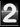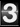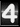Site Owner: Li Ke Xiong
Email: likexiong@126.com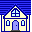The " Primary Proof " For Fermat Last Theorem

A. At the beginning, we think it is necessary to repeat our Definitions in the paper of " The Development of the Concept of Congruence "
Definition I (A).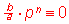mod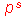. integer a, b, n and s.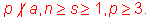(B). Never can "p" be as the denominator divisor of (and in) any finite expression,
otherwise meaningless.  (In any finite expressions, when "p" is a denominator divisor,
1/p is 1/0, hence the finite expression is meaningless, mod.
We should say, if A is a basic fact, B should be true, too. A and B can not be
separated.

B. Now, suppose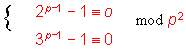can be true with same p.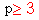We have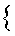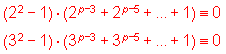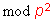,make the function f(x)=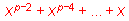the above two expressions are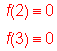, p>3.

C.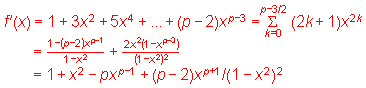D. Because(p>3)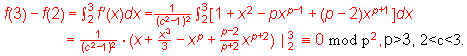E. Let us see the above (      )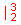, if (      )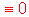p>3, surely (       )mod p.
if suppose (       )notmod p, (        )notwithout any doubt.
a. So let us suppose (      )mod p, we have (         )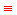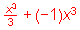,
please note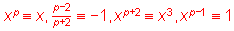when x is an integer,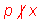mod p.
thus (      )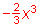= -2/3(27-8)=-2/3X19mod p (p>3)
The only possibility for (        )(p>3) is p=19.
b.Let us suppose (         )(p>3)
(        )=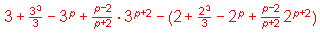due to having supposed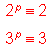so (         )3+9-3+(p-2)/(p+2)X27-[2+8/3-2+(p-2)/(p+2)X8]
=19[1/3+(p-2)/(p+2)](p>3)
1. If p=19, we get 1/3+(19-2)/(19+2)mod 19, we get 24,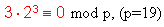or 1/3+(19-2)/(19+2)1/3+(-2)/2=1/3-1=(-2)/3mod 19.
2. If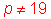, we get 1/3+(p-2)/(p+2), p>3 surely 1/3+(p-2)/(p+2),
that is 1/3-1mod p [Please note: (p-2)/(p+2)-1 ,pod p], so -2/3mod p, p>3
-2mod p, obviously this is also a contradiction.

F. Let us see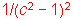,
a. Let us supposemod p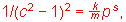k,m and s are integers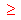1
s1,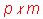. We know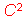should be a rational number, otherwise can not match
f(3)-f(2) is rational.  So we can write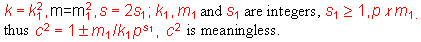b. Needless to sayis also a meaningless one.

G. From the above E and F, we can see f(3)-f(2) never. The conclusion is: there is
no such "p" to make, p>3 to be true together at the same time.Site Owner: Li Ke Xiong
Email: likexiong@126.com